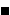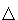# Cut The Knot!

An interactive column using Java applets
by Alex Bogomolny

January 2004What could be a natural framework for the following problem?

Prove that in bicentric quadrilaterals the incenter I, the circumcenter O and the point of intersection E of its diagonals are collinear.

The problem was proposed by India for, but not used in, the 1989 International Olympiad [Honsberger, p. 100]. It appeared in Crux Mathematicorum, 1989, 226 and also in the Russian Kvant, where it was solved by V. N. Dubrovsky using trigonometry. Ross Honsberger [Honsberger, p. 100-105] found another trigonometric solution based on a chapter 39 from [Dorrie] devoted to Fuss' problem (Find a relation between the radii and the distance beween the centers of the incircle and the circumcircle of a bicentric quadrilateral.)

Referring to its being submitted for a mathematical olympiad, Honsberger wrote: "I found this to be a very difficult problem. I have no idea how the proposers expected a contestant to approach the problem ..."

Below I paraphrase a note by A. Zaslavsky whose framework for the problem has rendered it virtually trivial. In all honesty, I can't imagine that the proposers might have had this approach in mind, for it requires even more insight into the nature of the problem than either of the trigonometric solutions. Nonetheless, in a hindsight, nothing could be more natural since, as a result, the problem is stripped of all its mystery.

So, instead of solving a specific problem concerning bicentric quadrilaterals, let's think of how they come into existence. How does one construct bicentric quadrilaterals? Here is one approach that fits so well the problem at hand.

Start with a quadrilateral ABCD. (Zaslavsky builds his theory for an arbitrary quadrilateral. In this column I shall assume ABCD cyclic. While not important, this assumption made writing an illustrative applet less messy. Which of course raises a legitimate question, Which comes first? Does the applet illustrate the theory, or the theory illustrates the applet?)

Assume the diagonals AC and BD of ABCD meet at P. Perpendiculars from P to the sides of ABCD determine a second quadrilateral KLMN [Honsberger, p. 60-64]. It's this quadrilateral KLMN we are going to be interested in. What makes KLMN bicentric? An answer emerges as a combination of two Propositions below.

### Propostition 1

KLMN is inscriptible.

### Proof

By construction, the quadrilateral KBLP is cyclic. Therefore,

 ∠KLP = ∠KBP,

as subtending the same chord KP. Note that ∠KBP = ∠ABD. Since LCMP is also cyclic, we similarly obtain

 ∠MLP = ∠MCP = ∠BCD.

For a cyclic quadrilateral ABCD, ∠ABD = ∠ACD, so that ∠KLP = ∠MLP. LP is therefore the bisector of angle L. Similarly KP, MP, and NP are bisectors of angles K, M, and N, respectively. Since they all meet in P, P is the incenter of KLMN.For KLMN to be bicentric, it must be made circumscriptable - cyclic. The condition for that is given by

### Proposition 2

KLMN is cyclic iff ABCD is orthodiagonal, i.e., iff AC ⊥ BD.

### Proof

Assume ABCD is orthodiagonal. By Brahmagupta, PK, PL, PM, and PN are maltitudes in quadrilateral ABCD, i.e., their extensions beyond P cross the opposite sides at their midpoints. Let those be K', L', M' and N'. The quadrilateral K'L'M'N' is none other than the Varignon parallelogram of the quadrilateral ABCD. Since the latter is orthodiagonal, K'L'M'N' is in fact a rectangle.

Rectangle is a cyclic shape with the circumcenter at the intersection of the diagonals, both of which are diameters of the incircle q. Now, for example, in ΔLL'N', angle L is right, while the hypotenuse L'N' is a diameter of circle q. L therefore lies on q. The same of course holds for K, M, and N.

To sum up, quadrilateral KLMN is inscribed in a circle centered at the intersection O of K'M' and L'N'.

Conversely, assume KLMN is cyclic and let's evaluate the angles inAPB. We'll show that ∠APB = 90°.

First of all, the cyclicity of KLMN is equivalent to

 (1) ∠LKN + ∠LMN = 180°.

By construction, four quadrilaterals MPND, MPLC, LPKB, and KPNA are also cyclic. From this we successively obtain

 (2) ∠NDP = ∠NMP, ∠LCP = ∠LMP, ∠LBP = ∠LKP, ∠NAP = ∠NKP.

Obviously

 (3) ∠NDP + ∠NAP = ∠CPD, ∠LBP + ∠LCP = ∠APB, ∠NMP + ∠LMP = ∠LMN, ∠LKP + ∠NKP = ∠LKN.

Together (2) and (3) lead to

 (4) ∠LKN + ∠LMN = ∠APB + ∠CPD = 2∠APB.

The required ∠APB = 90° now follows from (4) and (1).Let's recapitulate what we know of the quadrilateral KLMN when it's bicentric. Its incenter lies at the intersection of the diagonals of a "related" quadrilateral ABCD. Its circumcenter coincides with the intersection of two lines K'M' and L'N' whose endpoints lie on the sides of ABCD. The lines KK', LL', etc. meet in P, the intersection of the diagonals of ABCD. That's all that is needed to prove the collinearity of the three points P, E, and O.

### This applet requires Sun's Java VM 2 which your browser may perceive as a popup. Which it is not. If you want to see the applet work, visit Sun's website at https://www.java.com/en/download/index.jsp, download and install Java VM and enjoy the applet.

The assertion is indeed of projective nature. (This I believe justifies a little change in notations.) It is obviously true of parallelograms. Hence it is true of other quadrilaterals.

The problem has been solved for all bicentric quadrilaterals obtained from (cyclic) quadrilaterals ABCD by what Zaslavsky calls orthodiagonal mapping. But is it true that all bicentric quadrilaterals can be obtained in this manner? The answer is Yes, as it follows from the following

### Proposition 3

Let KLMN be bicentric. Through each of its vertices draw a line perpendicular to the line joining that vertex with its incenter P. The four lines form a cyclic quadrilateral ABCD with perpendicular diagonals that pass through P.

### Proof

By construction, PK, PL, etc. are the bisectors of the corresponding angles in KLMN. Angle counting in triangles KLP, LMP, MNP, and NKP gives

 (5) ∠KPN + ∠LPM = ∠KPL + ∠MPN.

Since the quadrilaterals MPND, MPLC, LPKB, and KPNA are cyclic, (5) implies

Each of the sums in (6) equals 180°. This shows that ABCD is a cyclic quadrilateral.

Let's now show that, say, APC is a straight line. From (2) and the fact that PK, PL, etc. are the angle bisectors in KLMN, we have

 (7) ∠KBP = ∠MCP, and ∠KAP = ∠MDP.

Comparing angles in triangles ABP and CDP and applying (7) gives

 (8) ∠APB = ∠CPD.

Similarly

 (9) ∠BPC = ∠APD,

from which it follows that ∠APC = 180°.### References

1. H. Dorrie, 100 Great Problems Of Elementary Mathematics, Dover Publications, NY, 1965
2. V. N. Dubrovsky, Solution to problem M1154, Kvant, n 8, 1989, pp 34-35 (in Russian), pdf is available at https://kvant.mccme.ru/1989/08/p34.htm.
3. R. Honsberger, In Pólya's Footsteps, MAA, 1999
4. A. A. Zaslavsky, The Orthodiagonal Mapping of Quadrilaterals, Kvant, n 4, 1998, pp 43-44 (in Russian), pdf is available at https://kvant.mccme.ru/1998/04/.•